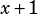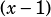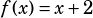I’m having a lot of trouble with this problem, could you please break it down for me? It is number 2 on the functions practice questions. “If f(x-1)=x+1 for all values of x, which of the following is equal to f(x+1)?”
A. x+3
B. x+2
C. x-1
D. x-3

In the solutions in the back of the book, I have two approaches. I’m assuming you’ve already looked at those and neither went all the way for you, so here’s another look.

We know that function notation means, generally, that the bit in parentheses goes through some series of mathematical operations, and a result is spit out. So, for example, stuff can be added or subtracted, or the argument could be multiplied, divided, raised to a power, etc.

In this particular problem, we know thatgoes through some process that spits out an end result of. Here’s the crux: what could happen toto make it equal? No multiplying or raising to powers necessary! The only thing you need to do to turnintois add 2!

That’s how we know that the function f just adds 2.Therefore, we can figure out whatmust be:Does that help?